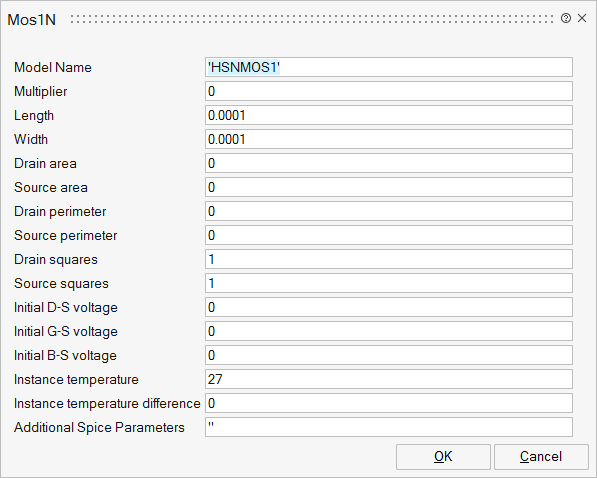# Mos1N

Level 1 MOSfet model with Meyer capacitance modelAnalog/Basic

## Description

This is a PMOS Level 1 (simple Schichman-Hodges Mosfet Model)

Proposed by Shockley, the equations of the MOS level 1 give the evaluation of the current IDS between the drain and the source as a function of VD, VG and VS.

The device operation is divided into three regions:

- cut-off (Vgs <= Vth)

- linear (0 <= Vds <= Vgs-Vth)

- saturated (0 <= Vgs-Vth <= Vds)

This model is only accurate for long channel.

Equations for the MOS1N device## ParametersNameLabelDescriptionData TypeValid Values

sp_mname

Model Name

Name of the NMOS model

String

sp_m

Multiplier

Multiplier for setting multiple mosfets in parallel

Scalar

sp_l

Length

Channel length (meter)

Scalar

sp_w

Width

Channel width (meter)

Scalar

Drain area

Drain Area (square meter)

Scalar

sp_as

Source area

Source area (square meter)

Scalar

sp_pd

Drain perimeter

Drain perimeter (square meter)

Scalar

sp_ps

Source perimeter

Source perimeter (square meter)

Scalar

sp_nrd

Drain squares

Equivalent number of squares of the drain diffusion

Scalar

sp_nrs

Source squares

Equivalent number of squares of the source diffusion

Scalar

sp_icvds

Initial D-S voltage

Initial drain source voltage (volts)

Scalar

sp_icvgs

Initial G-S voltage

Initial gate source voltage (volts)

Scalar

sp_icvbs

Initial B-S voltage

Initial bulk source voltage (volts)

Scalar

sp_temp

Instance temperature

The temperature at which the mosfet is operating (degrees Celsius)

Scalar

sp_dtemp

Instance temperature difference

Temperature variation relative to the simulation temperature (degrees Celsius)

Scalar

sp_Spice

For more details, see Additional Spice Parameters.

String

## Ports

NameTypeDescriptionIO TypeNumber

Drain

implicit

1

Gate

implicit

2

Source

implicit

3

Bulk

implicit

4